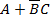# Assuming zero initial condition, the response y(t) of the system given below to a unit step input u(t) is

1.   u(t)

2.   tu(t)

3.  t2/2(t)

4.   e-tu(t)

4

tu(t)

Explanation :
No Explanation available for this question

# The minimized expression for the given K map (A: don’t care) is

1.

2.  B+AC

3.  C+AB

4.  ABC

4Explanation :
No Explanation available for this question

# Consider the following J-K flip-flop: In the given J-K flip-flop,   And K=1. Assume that the flip-flop was initially cleared and then clocked for 6 pulses. What is the sequence at the Q point

1.  010000

2.  011001

3.  010010

4.  010101

4

010101

Explanation :
No Explanation available for this question

# The  magnitude and phase transfer functions for a distortion less filter should respectively be

1.   (Magnitude)                                                (Phase)   Linear                                                         Constant

2.   (Magnitude)                                                (Phase)            Constant                                                Constant

3.   (Magnitude)                                                (Phase)   Constant                                                        Linear

4.   (Magnitude)                                                (Phase)   Linear                                                         Linear

4
 (Magnitude)                                                (Phase) Constant                                                        Linear

Explanation :
No Explanation available for this question

# The pole-zero pattern of a certain filter is shown in Fig.1. The filter must be of the following type

1.   Low –pass

2.  High-pass

3.  All-pass

4.  Band-pass

4

All-pass

Explanation :
No Explanation available for this question

# Let  , and h(t0 is a filter matched to g(t). If g(t) is applied as input to h(t), then the Fourier transform of the output is

1.

2.

3.

4.

4Explanation :
No Explanation available for this question

# A band limited signal is sampled at the Nyquist rate. The signal can be recovered by passing the samples through

1.   An RC filter

2.  An envelope detector

3.  A PLL

4.  An ideal low-pass filter with the appropriate bandwidth

4

An ideal low-pass filter with the appropriate bandwidth

Explanation :
No Explanation available for this question

# A band-limited signal with a maximum frequency of 5 khz is to be sampled According to the sampling theorem, the samplimg frequency which is not valid is

1.   5 kHz

2.  12 kHz

3.  15 kHz

4.  20 kHz

4

5 kHz

Explanation :
No Explanation available for this question

# A 4 GHz carrier is DSB_SC modulated by a low pass message signal with maximum frequency of 2 MHz. The resultant signal is to be ideally  samples. The minimum frequency of the sampling impulse train should be

1.  4 MHz

2.  8 MHz c.

3.   8 GHz

4.   8.004 GHz

4

8 MHz

c.

Explanation :
No Explanation available for this question

1.   (1/350)sec

2.  (π/350)sec

3.   (1/700)sec

4.  (π/175)sec

4